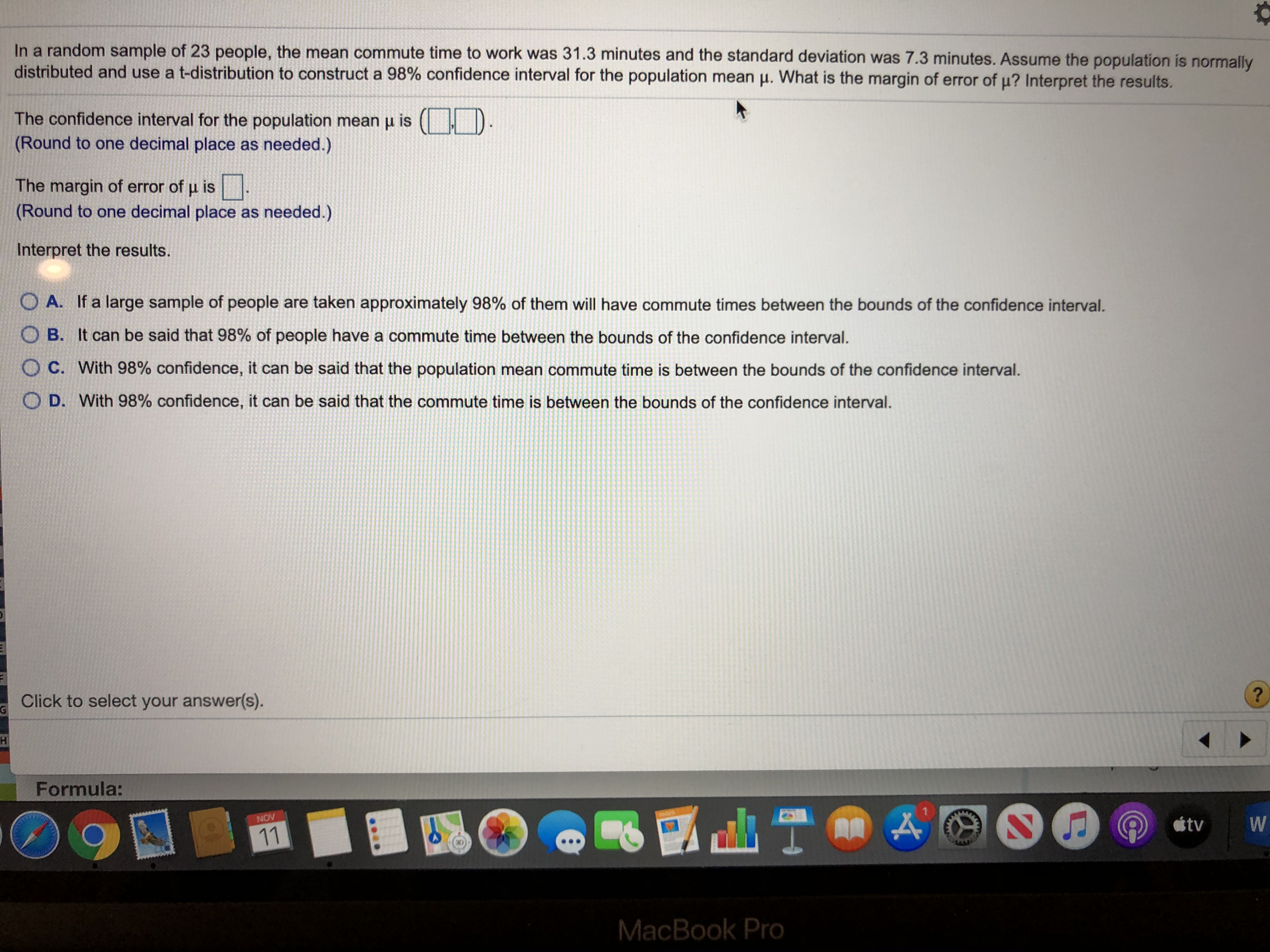# In a random sample of 23 people, the mean commute time to work was 31.3 minutes and the standard deviation was 7.3 minutes. Assume the population is normallydistributed and use a t-distribution to construct a 98% confidence interval for the population mean . What is the margin of error of u? Interpret the results.The confidence interval for the population mean u is ((Round to one decimal place as needed.)The margin of error of u is(Round to one decimal place as needed.)Interpret the results.O A. If a large sample of people are taken approximately 98% of them will have commute times between the bounds of the confidence interval.O B. It can be said that 98% of people have a commute time between the bounds of the confidence interval.O C. With 98% confidence, it can be said that the population mean commute time is between the bounds of the confidence interval.O D. With 98% confidence, it can be said that the commute time is between the bounds of the confidence interval.Click to select your answer(s).GHFormula:NOVWtv11MacBook Proo

Question
3 viewshelp_outlineImage TranscriptioncloseIn a random sample of 23 people, the mean commute time to work was 31.3 minutes and the standard deviation was 7.3 minutes. Assume the population is normally distributed and use a t-distribution to construct a 98% confidence interval for the population mean . What is the margin of error of u? Interpret the results. The confidence interval for the population mean u is ( (Round to one decimal place as needed.) The margin of error of u is (Round to one decimal place as needed.) Interpret the results. O A. If a large sample of people are taken approximately 98% of them will have commute times between the bounds of the confidence interval. O B. It can be said that 98% of people have a commute time between the bounds of the confidence interval. O C. With 98% confidence, it can be said that the population mean commute time is between the bounds of the confidence interval. O D. With 98% confidence, it can be said that the commute time is between the bounds of the confidence interval. Click to select your answer(s). G H Formula: NOV W tv 11 MacBook Pro o fullscreen
check_circle

Step 1

Calculating the confidence interval for population mean:

Sample mean = 31.3 minutes.

Sample standard deviation, s is 7.3 minutes.

The sample size, n is 23.

From the EXCEL, usin...

### Want to see the full answer?

See Solution

#### Want to see this answer and more?

Solutions are written by subject experts who are available 24/7. Questions are typically answered within 1 hour.*

See Solution
*Response times may vary by subject and question.
Tagged in

### Other